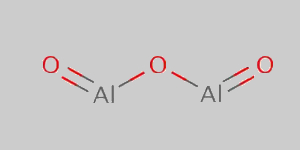# Aluminium Oxide Formula

Aluminium oxide formula is given here to help student easily learn and remember the formula during class tests or even in semester exams. Aluminium oxide is a chemical compound of oxygen and aluminium and occurs naturally in various minerals such as corundum, bauxite, etc. This compound is mostly amphoteric in nature and basically has chemical, industrial and commercial applications. Let us look at the formula below.

## Aluminium Oxide Chemical Formula

The formula of aluminium oxide is given as Al2O3. How is this formula generated? First of all, we have to consider that aluminium is a metal and Oxygen is a non-metal making it an ionic compound. There is some charge in the aluminium and oxygen ions. Aluminium has a +3 charge whereas oxygen has -2 charge. On applying the criss-cross method we get the formula of aluminium oxide. Usually, aluminium loses three electrons while oxygen gains two electrons in the chemical process and this is mainly done to achieve stability.

 Formula Al2O3 Molar Mass 101.960 g·mol−1 Density 3.987g/cm3 Melting Point 2,072 °C Boiling Point 2,977 °C

### Aluminium Oxide Structural Formula

Below is the diagram of the aluminium oxide structural formula.Students can check out the different formulas of important chemical compounds here at BYJU’S.